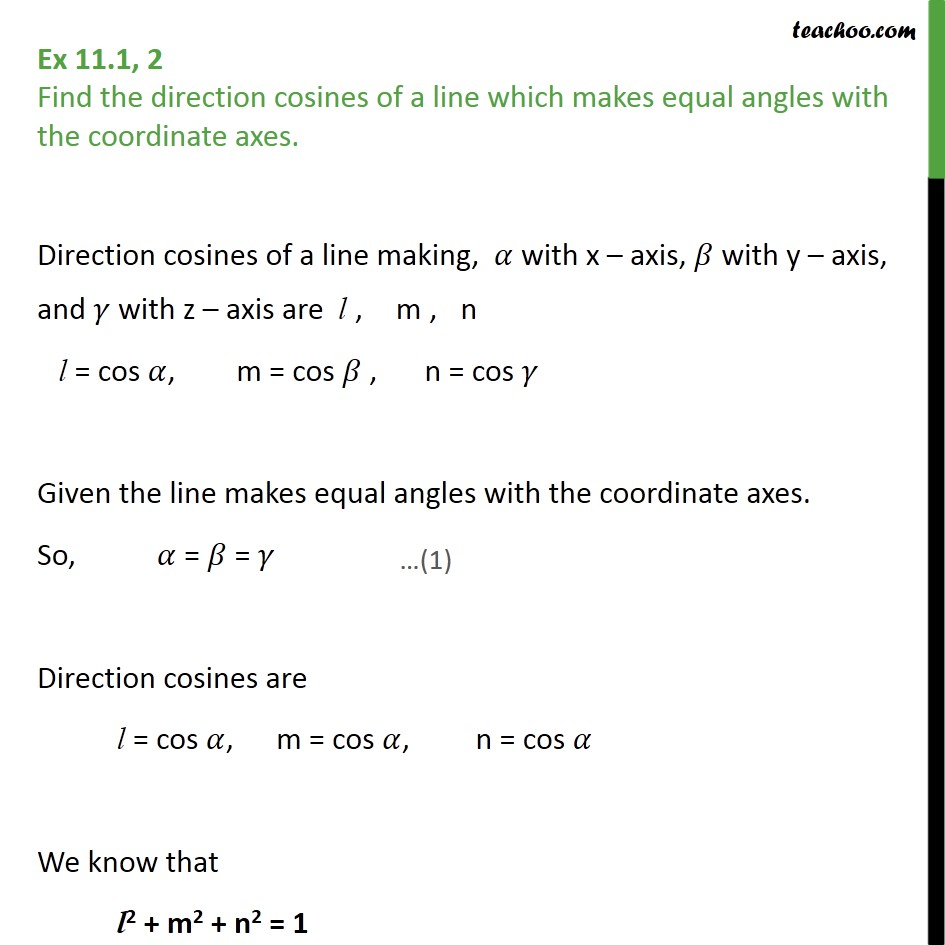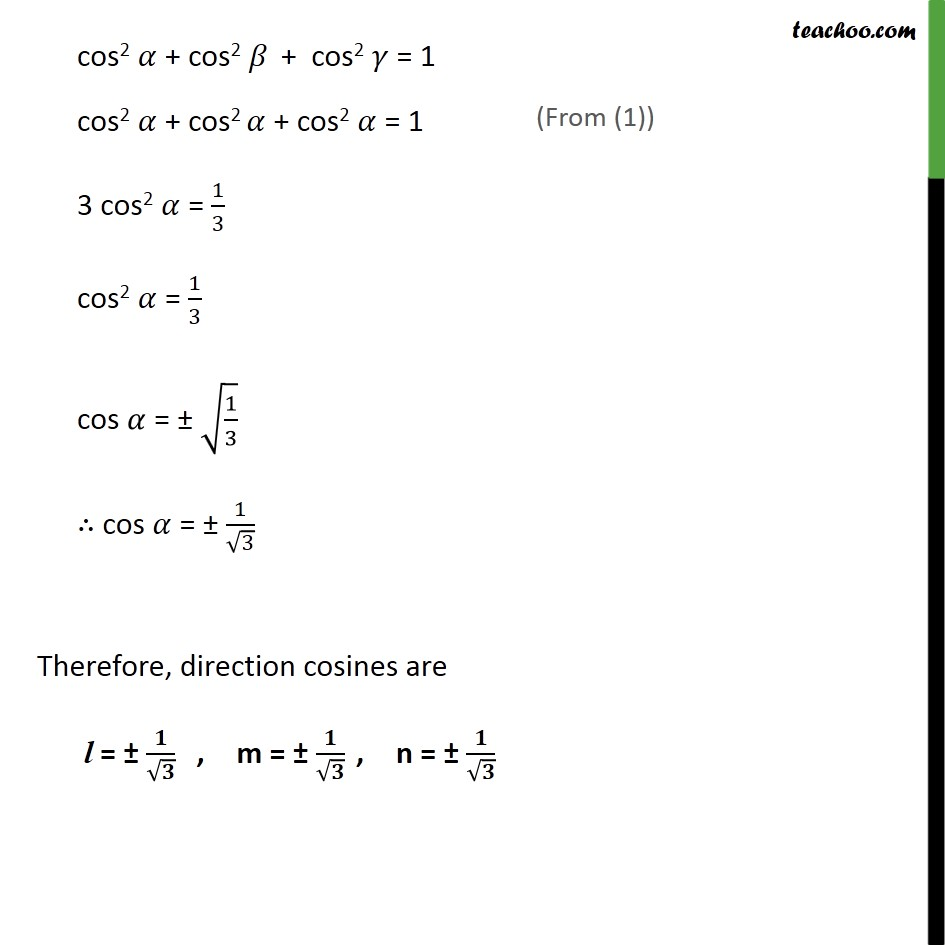1. Class 12
2. Important Question for exams Class 12
3. Chapter 11 Class 12 Three Dimensional Geometry

Transcript

Ex 11.1, 2 Find the direction cosines of a line which makes equal angles with the coordinate axes. Direction cosines of a line making, 𝛼 with x – axis, 𝛽 with y – axis, and 𝛾 with z – axis are l, m, n l = cos 𝛼, m = cos 𝛽 , n = cos 𝛾 Given the line makes equal angles with the coordinate axes. So, 𝛼 = 𝛽 = 𝛾 Direction cosines are l = cos 𝛼, m = cos 𝛼, n = cos 𝛼 We know that l2 + m2 + n2 = 1 cos2 𝛼 + cos2 𝛽 + cos2 𝛾 = 1 cos2 𝛼 + cos2 𝛼 + cos2 𝛼 = 1 3 cos2 𝛼 = 1﷮3﷯ cos2 𝛼 = 1﷮3﷯ cos 𝛼 = ± ﷮ 1﷮3﷯﷯ ∴ cos 𝛼 = ± 1﷮ ﷮3﷯﷯ Therefore, direction cosines are l = ± 𝟏﷮ ﷮𝟑﷯﷯ , m = ± 𝟏﷮ ﷮𝟑﷯﷯ , n = ± 𝟏﷮ ﷮𝟑﷯﷯

Chapter 11 Class 12 Three Dimensional Geometry

Class 12
Important Question for exams Class 12